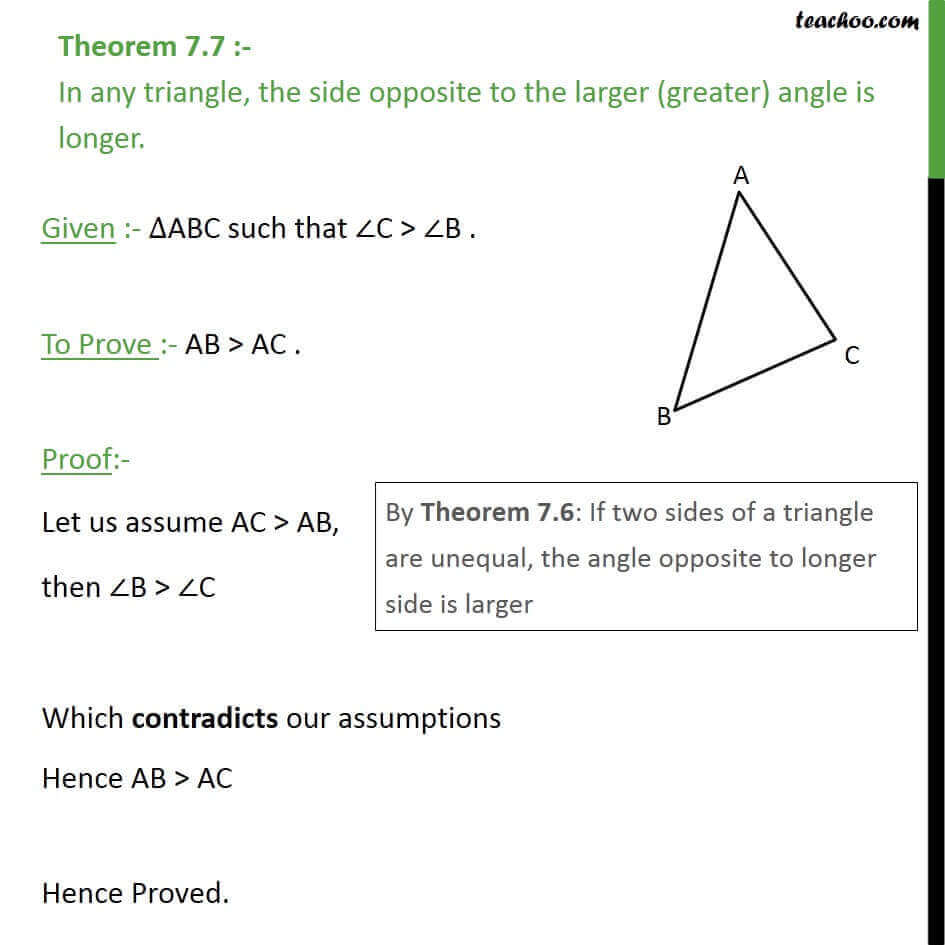1. Chapter 7 Class 9 Triangles (Term 1)
2. Serial order wise
3. Theorems

Transcript

Theorem 7.7 :- In any triangle, the side opposite to the larger (greater) angle is longer. Given :- ABC such that C > B . To Prove :- AB > AC . Proof:- Let us assume AC > AB, then B > C Which contradicts our assumptions Hence AB > AC Hence Proved.

Theorems